# Factoring PolynomialsPage 1

#### WATCH ALL SLIDES

Slide 1## Factoring Polynomials

Slide 2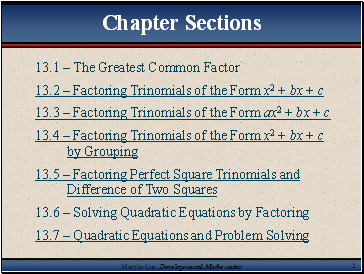13.1 – The Greatest Common Factor

13.2 – Factoring Trinomials of the Form x2 + bx + c

13.3 – Factoring Trinomials of the Form ax2 + bx + c

13.4 – Factoring Trinomials of the Form x2 + bx + c by Grouping

13.5 – Factoring Perfect Square Trinomials and Difference of Two Squares

13.6 – Solving Quadratic Equations by Factoring

13.7 – Quadratic Equations and Problem Solving

Chapter Sections

Slide 3## The Greatest Common Factor

Slide 4Factors

Factors (either numbers or polynomials)

When an integer is written as a product of integers, each of the integers in the product is a factor of the original number.

When a polynomial is written as a product of polynomials, each of the polynomials in the product is a factor of the original polynomial.

Factoring – writing a polynomial as a product of polynomials.

Slide 5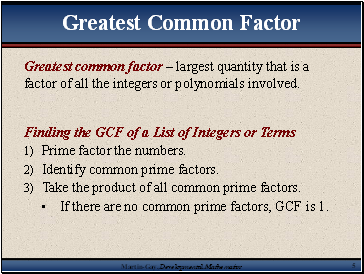Greatest common factor – largest quantity that is a factor of all the integers or polynomials involved.

Finding the GCF of a List of Integers or Terms

Prime factor the numbers.

Identify common prime factors.

Take the product of all common prime factors.

If there are no common prime factors, GCF is 1.

Greatest Common Factor

Slide 6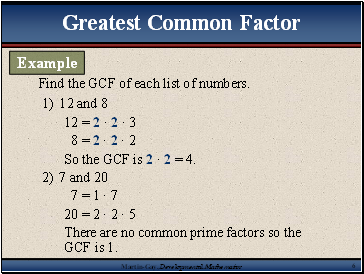Find the GCF of each list of numbers.

12 and 8

12 = 2 · 2 · 3

8 = 2 · 2 · 2

So the GCF is 2 · 2 = 4.

7 and 20

7 = 1 · 7

20 = 2 · 2 · 5

There are no common prime factors so the GCF is 1.

Greatest Common Factor

Example

Slide 7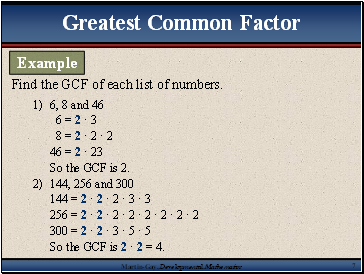Find the GCF of each list of numbers.

6, 8 and 46

6 = 2 · 3

8 = 2 · 2 · 2

46 = 2 · 23

So the GCF is 2.

144, 256 and 300

144 = 2 · 2 · 2 · 3 · 3

256 = 2 · 2 · 2 · 2 · 2 · 2 · 2 · 2

300 = 2 · 2 · 3 · 5 · 5

So the GCF is 2 · 2 = 4.

Greatest Common Factor

Example

Slide 8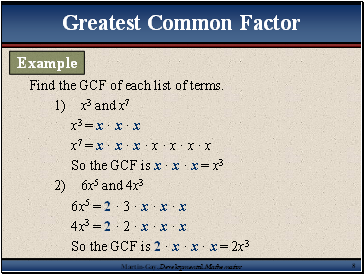x3 and x7

x3 = x · x · x

x7 = x · x · x · x · x · x · x

So the GCF is x · x · x = x3

6x5 and 4x3

6x5 = 2 · 3 · x · x · x

4x3 = 2 · 2 · x · x · x

So the GCF is 2 · x · x · x = 2x3

Find the GCF of each list of terms.

Greatest Common Factor

Example

Slide 9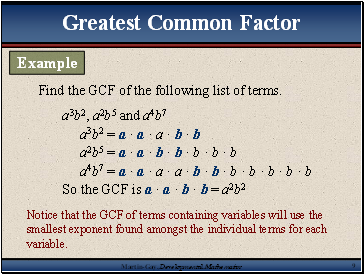Go to page:
1  2  3  4  5  6  7  8  9  10